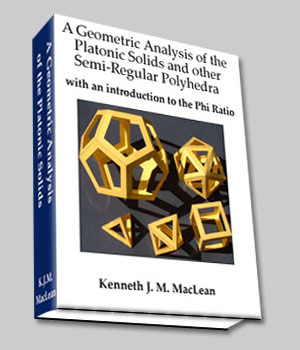The Fibonacci Series

Because division or growth by the Golden Mean has no beginning or ending, it is not a good candidate for constructing forms of any kind.  It is necessary to have a definable starting point if you want to build anything.

However, there is an approximation to the Golden Mean that nature uses, called the Fibonacci Sequence.  Leonardo Fibonacci was a monk who noticed that branches on  trees, leaves on flowers, and seeds in pine cones and sunflower seeds arranged themselves in this sequence.
The Fibonacci Sequence is based on the golden mean ratio, or Phi ().  To the left of the equal sign is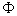raised to a power, and to the right of the equal sign is the Fibonacci Sequence: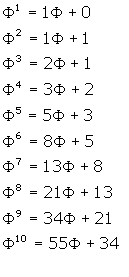...
Each digit in the second column to the left of thesymbol is the sum of the 2 before it in the previous row:
1 + 0 = 1, 1 + 1 = 2, 2+1 = 3, 3 + 2 = 5, 5 + 3 = 8, ..... and so on.

Notice that when one divides the digit by the one before it in the sequence, the result approaches Ø:

1 / 1   = 1.0
2 / 1   = 2.0
3 / 2   = 1.5
5 / 3   = 1.67
8 / 5   = 1.60
13 / 8 = 1.625
21 / 13 = 1.6153846
34 / 21 = 1.6190476
55 / 34 =  1.617647
...
Here is a chart that shows this:Notice thatis approached both from above and below.  This is not an asymptotic approach, but one which affords a full view of both sides. Like a signal locking in on the target, the Fibonacci sequence homes in on.
Theratio is attained from the division of integers. Integers, or whole numbers, represent things that can be designed and built in the 'real world.' Even thoughis unattainable, nature can closely approximate it, as we can see from the following photographs:A Nautilus shell    (1)Echeveria Agavoides (2)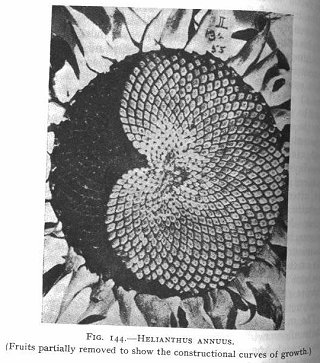Helianthus Annus  (2)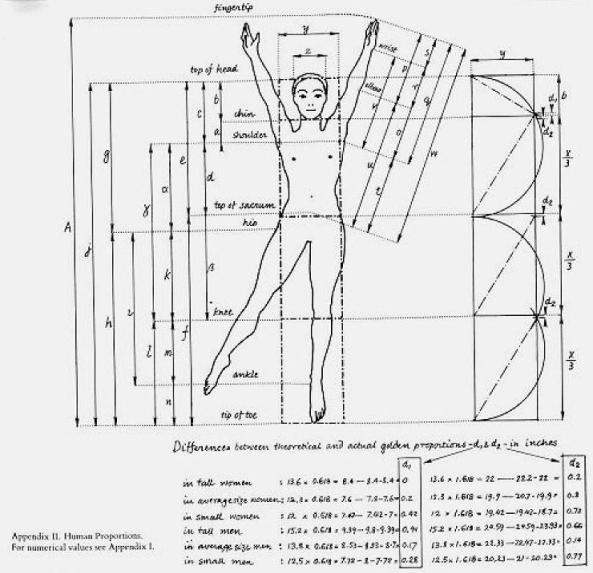human (3)

The human body has many Phi/Fibonacci relationships.

The leg: The distance from the hip to the knee, and from the knee to the ankle.
The face: The distance from the top of the head to the nose, and from the nose to the chin.
The arm: The distance between the shoulder joint to the elbow, and from the elbow to the finger tips.
The hand: The distance between the wrist, the knuckles, the first and second joints of the fingers, and the finger tips.

Of course every body is different and these relationships are only approximate. Once you become aware of the Fibonacci sequence / Phi ratio, however, you begin to see it in many life forms.

(As an aside, note that in the Fibonacci sequence, you don't have to start with 1. Begin with any number and proceed
with the rule "the current number is the sum of the 2 previous numbers." Do the division of the current number by the prior number in the sequence andwill always be the result.)

On to The Properties of Phi
_______________________________________________
(1) The Geometry of Art and Life    -- Matila Ghyka
(2) The Curves of Life --  Theodore Andreas Cook
(3) The Power Of Limits --  Gyorgy Doczi